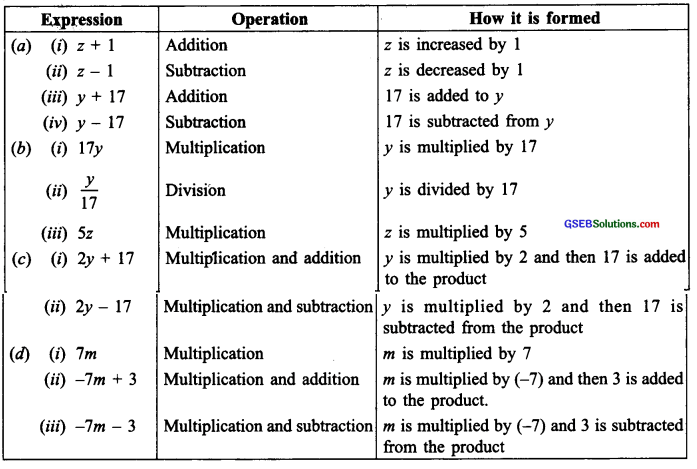# GSEB Solutions Class 6 Maths Chapter 11 Algebra Ex 11.3

Gujarat Board GSEB Textbook Solutions Class 6 Maths Chapter 11 Algebra Ex 11.3 Textbook Questions and Answers.

## Gujarat Board Textbook Solutions Class 6 Maths Chapter 11 Algebra Ex 11.3

Question 1.
Makeup as many expressions with numbers {no variables) as you can from three numbers 5, 7, and 8. Every number should be used not more than once. Use only addition, subtraction, and multiplication.
Hint: Three possible expressions are 5 + (8 – 7), 5 – (8 – 7), (5 x 8) + 7; make the other expressions.
Solution:
Here, we have to use the given three numbers altogether. In an expression, every number is not to be used more than once.
Thus, we can have:
(i) 5 + (7 + 8)
(ii) 7 × 5 + 8
(iii) (8 – 5) × 7
(iv) (7 – 5) × 8
(v) (5 × 7) – 8
(vi) (8 × 7) + 5
(vii) 8 – 5 + 7
(viii) (8 – 7) + 5, etc.Question 2.
Which out of the following are expressions with numbers only?
(a) y + 3
(b) (7 × 20) – 8z
(c) 5(21 -7) + 7 × 2
(d) 5
(e) 3x
(f) 5 – 5n
(g) (7 × 20) – (5 × 10) – 45 + p
Solution:
In expressions (c) and (d) no variable is involved.
∵ (c) and (d) are expressions with numbers only.Question 3.
Identify the operations (addition, subtraction, division, multiplication) informing the following expressions and tell how the expressions have been formed.
(a) z + 1, z – l, y + 17, y – 17
(b) 17y, $$\frac{y}{17}$$, 5z
(c) 2y + 17, 2y – 17
(d) 7m, – 7m + 3, – 7m – 3
Solution:Question 4.
Give expressions for the following cases.
(b) 7 subtracted from p
(c) p multiplied by 7
(d) p divided by 7
(e) 7 subtracted from -m
(f) -p multiplied by 5
(g) -p divided by 5
(h) p multiplied by -5
Solution:
(a) P + 7
(b) p – 7
(c) 7p
(d) $$\frac{p}{7}$$
(e) (-m) -7
(f) 5 x (-p) or -5p
(g) $$\frac{-p}{5}$$
(h) -5pQuestion 5.
Give expressions in the following cases.
(b) 11 subtracted from 2m
(c) 5 times y to which 3 is added
(d) 5 times y from which 3 is subtracted
(e) y is multiplied by -8
(f) y is multiplied by -8 and then 5 is added to the result
(g) y is multiplied by 5 and the result is subtracted from 16
(h) y is multiplied by -5 and the result is added to 16.
Solution:
(a) 2m + 11
(b) 2m – 11
(c) (5xy) + 3 = 5y + 3
(d) (5 x y) – 3 = 5y – 3
(e) y x (-8) = -8y
(f) [y x (-8)] + 5 = -8y + 5
(g) 16 – (y x 5) = 16 – 5y
(h) 16 + [y x (-5)] = 16 + (-5y)Question 6.
(a) Form expressions using t and 4. Use not more than one number operation. Every expression must have t in it.
(b) Form expressions using y, 2, and 7. Every expression must have in it. Use only two number operations. These should be different.
Solution:
(a) We can have the following expressions:
t + 4, t – 4, 4t, $$\frac{t}{4}$$ , 4 – t…
(b) 2y + 7, 2y – 7, 7y + 2, 7y – 2…